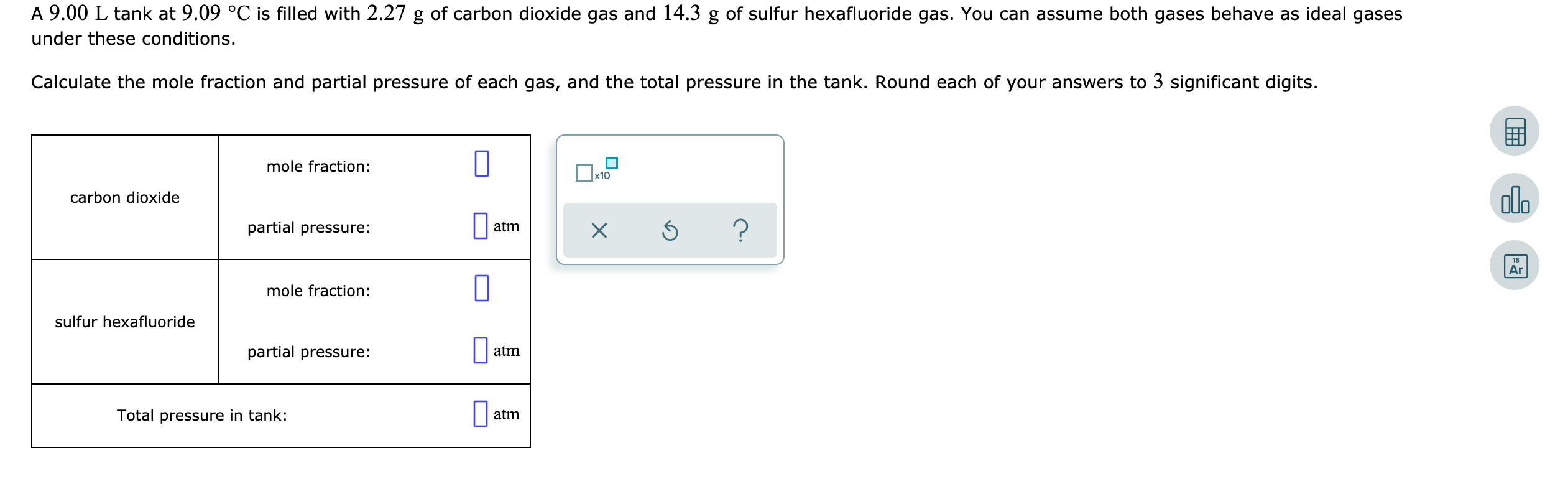Home / Answered Questions / Other / a-9-00-l-tank-at-9-09-c-is-filled-with-2-27-g-of-carbon-dioxide-gas-and-14-3-g-of-sulfur-hexafluorid-aw228

# (Solved): A 9.00 L Tank At 9.09 Â°C Is Filled With 2.27 G Of Carbon Dioxide Gas And 14.3 G Of Sulfur Hexafluor...A 9.00 L tank at 9.09 Â°C is filled with 2.27 g of carbon dioxide gas and 14.3 g of sulfur hexafluoride gas. You can assume both gases behave as ideal gases under these conditions. Calculate the mole fraction and partial pressure of each gas, and the total pressure in the tank. Round each of your answers to 3 significant digits. mole fraction: carbon dioxide partial pressure: atmx ? mole fraction: sulfur hexafluoride partial pressure: atm Total pressure in tank: atm

We have an Answer from Expert